# OmnesAudio

Omnes Audio develops speaker for car hifi, multimedia and home stereo systems.

## Tweeter## OmnesAudio AMT 50

order no. BP-AMT50

EUR 45,00excl. VAT: € 37.82 / \$ 40.84

.

• impedance R = 4 Ohm## OmnesAudio T25 H

order no. BP-T25H

EUR 18,00excl. VAT: € 15.13 / \$ 16.34

.

• power handling (continuous/programme) = 40 W
• resonance frequency fs = 900 Hz
• impedance R = 6 Ohm
• sound pressure level SPL = 92 dB (2,83V; 1m)
• DC resistance Re = 5,6 Ohm
• total Q factor Qts = 1,03 (Qms=2,39, Qes=1,81)
• mounting diameter d = 43 mm
• overall diameter d = 70 mm
• mounting depth (not countersunk) t = 24 mm
PDF data sheet

## Full Range## OmnesAudio BB 2.5 AL

order no. BP-BB25al

EUR 14,00excl. VAT: € 11.76 / \$ 12.71

.

• resonance frequency fs = 172 Hz
• impedance R = 4 Ohm
• sound pressure level SPL = 82 dB (2,83V; 1m)
• sound pressure level SPL = dB (1W; 1m)
• DC resistance Re = 3,5 Ohm
• force factor BL = 2,3 N/A
• equivalent volume of compliance Vas = 0,18 l
• total Q factor Qts = 0,73 (Qms=3,31, Qes=0,94)
• voice coil diameter = 20,4 mm
• maximum peak linear excursion vibration xlin = +/- 1,5 mm
• mass m = 75 kgrecommended cabinet 1:
closed cabinet with 0,91 L volume
from 128/81 Hz (-3dB/-8dB)

recommended cabinet 2:
3,7 L volume bass reflex cabinet
with HP35 reflex tube, 14 cm long.
from 60/50 Hz (-3dB/-8dB).

## OmnesAudio BB 3.AL

order no. BP-BB3al

EUR 42,00excl. VAT: € 35.29 / \$ 38.12

.

• power handling (continuous/programme) = 30 W
• frequency range = 76-18000 Hz
• resonance frequency fs = 76 Hz
• impedance R = 8 Ohm
• sound pressure level SPL = 84 dB (2,83V; 1m)
• DC resistance Re = 6,6 Ohm
• force factor BL = 4,18 N/A
• effective piston radiating area Sd = 31 cm2
• equivalent volume of compliance Vas = 1,99 l
• total Q factor Qts = 0,48 (Qms=4,8, Qes=0,53)
• voice coil diameter = 25 mm
• maximum peak linear excursion vibration xlin = +/- 2 mm
• mounting diameter d = 77,5 mm
• overall diameter d = 98 mm
• mounting depth (not countersunk) t = 47 mm
PDF data sheetrecommended cabinet 1:
closed cabinet with 0,64 L volume
from 156/98 Hz (-3dB/-8dB)

recommended cabinet 2:
2,5 L volume bass reflex cabinet
with 2cm-Rohr reflex tube, 5 cm long.
from 75/61 Hz (-3dB/-8dB).

## OmnesAudio BB 3.01A

order no. BP-BB301A- not available

.

• power handling (continuous/programme) = 30 W
• frequency range = 100-20000 Hz
• resonance frequency fs = 85 Hz
• impedance R = 4 Ohm
• sound pressure level SPL = 86 dB (2,83V; 1m)
• force factor BL = 2,89 N/A
• effective piston radiating area Sd = 29 cm2
• equivalent volume of compliance Vas = 1,8 l
• total Q factor Qts = 0,43 (Qms=2,2, Qes=0,53)
• mounting diameter d = 74 mm
• overall diameter d = 93 mm
• mounting depth (not countersunk) t = 45 mm
PDF data sheet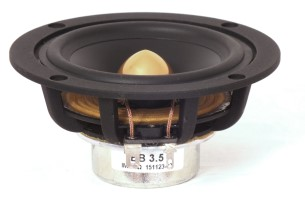## OmnesAudio BB 3.5

order no. BP-BB35

EUR 38,00excl. VAT: € 31.93 / \$ 34.49

.

• power handling (continuous/programme) = 30 W
• frequency range = 120-20000 Hz
• resonance frequency fs = 110 Hz
• impedance R = 8 Ohm
• sound pressure level SPL = 85 dB (2,83V; 1m)
• DC resistance Re = 6,8 Ohm
• force factor BL = 4,3 N/A
• effective piston radiating area Sd = 38,5 cm2
• equivalent volume of compliance Vas = 1,29 l
• total Q factor Qts = 0,72 (Qms=6,26, Qes=0,813)
• voice coil diameter = 25 mm
• maximum peak linear excursion vibration xlin = +/- 2,2 mm
• mounting diameter d = 88 mm
• overall diameter d = 104 mm
• mounting depth (not countersunk) t = 50 mm
PDF data sheet

## Coaxial speakerrecommended cabinet 1:
closed cabinet with 0,71 L volume
from 221/139 Hz (-3dB/-8dB)

recommended cabinet 2:
2,9 L volume bass reflex cabinet
with HP50 reflex tube, 11 cm long.
from 101/86 Hz (-3dB/-8dB).

## OmnesAudio CX 3.1

order no. BP-CX30

EUR 55,00excl. VAT: € 46.22 / \$ 49.92

10 cm coax driver with aluminium cone.

• frequency range = 200-20000 Hz
• resonance frequency fs = 142 Hz
• impedance R = 8 Ohm
• sound pressure level SPL = 84 dB (2,83V; 1m)
• DC resistance Re = 6 Ohm
• force factor BL = 4,96 N/A
• effective piston radiating area Sd = 48 cm2
• effective mechanical mass incl. air load mms = 3,1 g
• equivalent volume of compliance Vas = 1,2 l
• total Q factor Qts = 0,52 (Qms=2,51, Qes=0,65)
• voice coil diameter = 30 mm
• mounting diameter d = 87 mm
• overall diameter d = 116 mm
• mounting depth (not countersunk) t = 60 mm
PDF data sheetrecommended cabinet 1:
closed cabinet with 0,71 L volume
from 221/139 Hz (-3dB/-8dB)

recommended cabinet 2:
2,9 L volume bass reflex cabinet
with HP50 reflex tube, 11 cm long.
from 101/86 Hz (-3dB/-8dB).

## OmnesAudio CX 3.3

order no. BP-CX33

EUR 55,00excl. VAT: € 46.22 / \$ 49.92

10 cm coax driver with aluminium cone.

• frequency range = 200-20000 Hz
• resonance frequency fs = 142 Hz
• impedance R = 8 Ohm
• sound pressure level SPL = 84 dB (2,83V; 1m)
• DC resistance Re = 6 Ohm
• force factor BL = 4,96 N/A
• effective piston radiating area Sd = 48 cm2
• effective mechanical mass incl. air load mms = 3,1 g
• equivalent volume of compliance Vas = 1,2 l
• total Q factor Qts = 0,52 (Qms=2,51, Qes=0,65)
• voice coil diameter = 30 mm
• mounting diameter d = 87 mm
• overall diameter d = 116 mm
• mounting depth (not countersunk) t = 60 mm
PDF data sheet

## Wooferrecommended cabinet 1:
closed cabinet with 2,9 L volume
from 115/73 Hz (-3dB/-8dB)

recommended cabinet 2:
11,2 L volume bass reflex cabinet
with HP70 reflex tube, 22 cm long.
from 56/46 Hz (-3dB/-8dB).

## OmnesAudio MW 5.0 Alu

order no. BP-mw50alu

EUR 28,00excl. VAT: € 23.53 / \$ 25.41

.

• power handling (continuous/programme) = 70 W
• frequency range = 60- Hz
• resonance frequency fs = 60 Hz
• impedance R = 4 Ohm
• sound pressure level SPL = 88 dB (2,83V; 1m)
• DC resistance Re = 3,6 Ohm
• force factor BL = 5,2 N/A
• effective piston radiating area Sd = 95 cm2
• effective mechanical mass incl. air load mms = 9,49 g
• equivalent volume of compliance Vas = 9,34 l
• total Q factor Qts = 0,41 (Qms=2,97, Qes=0,47)
• voice coil diameter = 25 mm
• maximum peak linear excursion vibration xlin = +/- 3 mm
• mounting diameter d = 127 mm
• overall diameter d = 152 mm
• mounting depth (not countersunk) t = 67 mm
PDF data sheetrecommended cabinet 1:
closed cabinet with 6,1 L volume
from 82/52 Hz (-3dB/-8dB)

recommended cabinet 2:
24 L volume bass reflex cabinet
with HP70 reflex tube, 19 cm long.
from 40/33 Hz (-3dB/-8dB).

## OmnesAudio MW6 B

order no. BP-mw6b

EUR 25,00excl. VAT: € 21.01 / \$ 22.69

.

• resonance frequency fs = 45 Hz
• impedance R = 8 Ohm
• sound pressure level SPL = 89,8443 dB (2,83V; 1m)
• DC resistance Re = 6,9 Ohm
• force factor BL = 7,6761 N/A
• effective piston radiating area Sd = 134 cm2
• effective mechanical mass incl. air load mms = 14,3096 g
• equivalent volume of compliance Vas = 17,229 l
• total Q factor Qts = 0,44 (Qms=2,5119, Qes=0,5335)
• voice coil diameter = 32 mm
• maximum peak linear excursion vibration xlin = +/- 4,1 mm
• mounting diameter d = 142 mm
• overall diameter d = 187 mm
• mounting depth (not countersunk) t = 92 mmrecommended cabinet 1:
closed cabinet with 7,5 L volume
from 70/44 Hz (-3dB/-8dB)

recommended cabinet 2:
30 L volume bass reflex cabinet
with HP70 reflex tube, 23 cm long.
from 34/27 Hz (-3dB/-8dB).

## OmnesAudio SW 8.01 ehemals W8-670Z

order no. BP-W8-670Z

EUR 48,00excl. VAT: € 40.34 / \$ 43.56

.

• power handling (continuous/programme) = 100 W
• resonance frequency fs = 38 Hz
• impedance R = 4 Ohm
• sound pressure level SPL = 85 dB (2,83V; 1m)
• force factor BL = 9,07 N/A
• effective piston radiating area Sd = 209 cm2
• effective mechanical mass incl. air load mms = 49,95 g
• equivalent volume of compliance Vas = 21,2 l
• total Q factor Qts = 0,42 (Qms=3,86, Qes=0,47)
• maximum peak linear excursion vibration xlin = +/- 12 mm
• mounting diameter d = 184 mm
• overall diameter d = 204,5 mm
• mounting depth (not countersunk) t = 87 mm
PDF data sheet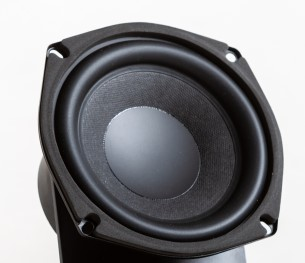## OmnesAudio SW 5.01

order no. BP-SW501

EUR 36,00excl. VAT: € 30.25 / \$ 32.67

.

• resonance frequency fs = 47,61 Hz
• DC resistance Re = 3,8 Ohm
• force factor BL = 7,88 N/A
• effective piston radiating area Sd = 86,5901 cm2
• effective mechanical mass incl. air load mms = 25,4913 g
• equivalent volume of compliance Vas = 4,59 l
• total Q factor Qts = 0,43 (Qms=5,31, Qes=0,47)
• maximum peak linear excursion vibration xlin = +/- 6 mm

## no longer sold## OmnesAudio BB 8.01

order no. not-available- not available

.## OmnesAudio AMT 60.1

order no. not-available- not available

.

• power handling (continuous/programme) = 80 W
• frequency range = 2000-30000 Hz
• impedance R = 6 Ohm
• sound pressure level SPL = 91 dB (2,83V; 1m)
• DC resistance Re = 6 Ohm
• effective piston radiating area Sd = 84,94 cm2
• mounting diameter d = 60,6x82,3 mm
• overall diameter d = 110 mm
• mounting depth (not countersunk) t = 28 mm
PDF data sheet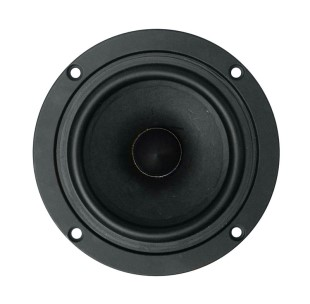## OmnesAudio BB 4 black

order no. not-available- not available

.

• resonance frequency fs = 75 Hz
• impedance R = 4 Ohm
• sound pressure level SPL = 86,85 dB (2,83V; 1m)
• DC resistance Re = 3,4 Ohm
• force factor BL = 3,496 N/A
• effective piston radiating area Sd = 53 cm2
• equivalent volume of compliance Vas = 4 l
• total Q factor Qts = 0,46 (Qms=2,234, Qes=0,581)

## OmnesAudio AMT 21 S BP 1.1

order no. not-available- not available

air motion transformer.

## OmnesAudio AMT 25 S BP 2.1

order no. not-available- not available

air motion transformer.

• power handling (continuous/programme) = 25 W
• frequency range = 1100-32000 Hz
• impedance R = 7 Ohm
• sound pressure level SPL = 91 dB (2,83V; 1m)recommended cabinet 1:
closed cabinet with 0,71 L volume
from 163/103 Hz (-3dB/-8dB)

recommended cabinet 2:
2,9 L volume bass reflex cabinet
with HP35 reflex tube, 10 cm long.
from 76/64 Hz (-3dB/-8dB).

## OmnesAudio BB 3.01

order no. not-available- not available

8 cm full range driver with paper cone. The magnet system is based on Neodymium technology.

• power handling (continuous/programme) = 15/40 W
• frequency range = 100-20000 Hz
• resonance frequency fs = 97 Hz
• impedance R = 4 Ohm
• sound pressure level SPL = 86 dB (2,83V; 1m)
• DC resistance Re = 3,5 Ohm
• force factor BL = 2,89 N/A
• voice coil inductance L = 0,07 mH
• effective piston radiating area Sd = 31,1 cm2
• effective mechanical mass incl. air load mms = 2,33 g
• equivalent volume of compliance Vas = 1,56 l
• total Q factor Qts = 0,46 (Qms=2,52, Qes=0,56)
• mounting diameter d = 74 mm
• overall diameter d = 93 mm
• mounting depth (not countersunk) t = 45 mm
PDF data sheet

## OmnesAudio T27

order no. not-available- not available

25 mm dome tweeter with fabric dome.

• frequency range = 1000-30000 Hz
• resonance frequency fs = 650 Hz
• impedance R = 4 Ohm
• sound pressure level SPL = 93,5 dB (2,83V; 1m)
• DC resistance Re = 2,75 Ohm
• force factor BL = 2,2 N/A
• effective piston radiating area Sd = 7 cm2
• effective mechanical mass incl. air load mms = 0,42 g
• total Q factor Qts = 0,67 (Qms=4,32, Qes=0,79)
• voice coil diameter = 25 mm
• mounting diameter d = 72 mm
• overall diameter d = 104 mm
• mounting depth (not countersunk) t = 25,4 mm
PDF data sheet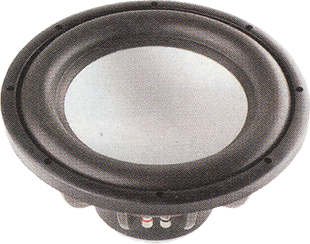recommended cabinet 1:
closed cabinet with 17 L volume
from 56/35 Hz (-3dB/-8dB)

recommended cabinet 2:
69 L volume bass reflex cabinet
with HP100 reflex tube, 33 cm long.
from 26/22 Hz (-3dB/-8dB).

## OmnesAudio SW 12.01 AC

order no. not-available- not available

31 cm bass driver with aluminium cone.

• power handling (continuous/programme) = 350/600 W
• frequency range = 32-500 Hz
• resonance frequency fs = 31,8 Hz
• impedance R = 4 Ohm
• sound pressure level SPL = 90,5 dB (2,83V; 1m)
• DC resistance Re = 3,2 Ohm
• force factor BL = 14,9 N/A
• voice coil inductance L = 0,49 mH
• effective piston radiating area Sd = 479 cm2
• effective mechanical mass incl. air load mms = 186 g
• equivalent volume of compliance Vas = 42,8 l
• total Q factor Qts = 0,44 (Qms=5,57, Qes=0,48)
• mounting diameter d = 285 mm
• overall diameter d = 325 mm
• mounting depth (not countersunk) t = 155 mmrecommended cabinet 1:
closed cabinet with 12,7 L volume
from 72/46 Hz (-3dB/-8dB)

recommended cabinet 2:
43 L volume bass reflex cabinet
with HP100 reflex tube, 33 cm long.
from 38/30 Hz (-3dB/-8dB).

## OmnesAudio SW 12.01 PC

order no. not-available- not available

31 cm bass driver with paper cone.

• power handling (continuous/programme) = 350/600 W
• frequency range = 32-500 Hz
• resonance frequency fs = 31,6 Hz
• impedance R = 4 Ohm
• sound pressure level SPL = 89,4 dB (2,83V; 1m)
• DC resistance Re = 3,2 Ohm
• force factor BL = 15,1 N/A
• voice coil inductance L = 2,39 mH
• effective piston radiating area Sd = 479 cm2
• effective mechanical mass incl. air load mms = 126 g
• equivalent volume of compliance Vas = 64,3 l
• total Q factor Qts = 0,34 (Qms=9,49, Qes=0,35)
• voice coil diameter = 63 mm
• maximum peak linear excursion vibration xlin = +/- 12 mm
• mounting diameter d = 285 mm
• overall diameter d = 325 mm
• mounting depth (not countersunk) t = 155 mm
PDF data sheetrecommended cabinet 1:
closed cabinet with 11,5 L volume
from 80/50 Hz (-3dB/-8dB)

recommended cabinet 2:
46 L volume bass reflex cabinet
with HP70 reflex tube, 9 cm long.
from 36/31 Hz (-3dB/-8dB).

## OmnesAudio MW 5W

order no. not-available- not available

14 cm bass-midrange driver with paper cone. To avoid compression effects the driver possesses a pole piece hole. The spider is rear ventilated for better cooling.

• frequency range = 60-6000 Hz
• resonance frequency fs = 56 Hz
• impedance R = 6 Ohm
• sound pressure level SPL = 88 dB (2,83V; 1m)
• DC resistance Re = 5,24 Ohm
• force factor BL = 4,53 N/A
• effective piston radiating area Sd = 95 cm2
• effective mechanical mass incl. air load mms = 7,2 g
• equivalent volume of compliance Vas = 14 l
• total Q factor Qts = 0,56 (Qms=3,85, Qes=0,65)
• voice coil diameter = 25,55 mm
• mounting diameter d = 124 mm
• overall diameter d = 150 mm
• mounting depth (not countersunk) t = 71 mm
PDF data sheetopen a bigger photo

recommended cabinet 1:
closed cabinet with 7,7 L volume
from 88/56 Hz (-3dB/-8dB)

recommended cabinet 2:
29 L volume bass reflex cabinet
with HP70 reflex tube, 13 cm long.
from 44/35 Hz (-3dB/-8dB).

## OmnesAudio MW 6B

order no. not-available- not available

16 cm bass-midrange driver with paper cone. To avoid compression effects the driver possesses a pole piece hole. The spider is rear ventilated for better cooling.

• frequency range = 50-5000 Hz
• resonance frequency fs = 44 Hz
• impedance R = 8 Ohm
• sound pressure level SPL = 87 dB (2,83V; 1m)
• DC resistance Re = 6,3 Ohm
• force factor BL = 7 N/A
• effective piston radiating area Sd = 135 cm2
• effective mechanical mass incl. air load mms = 12 g
• equivalent volume of compliance Vas = 28 l
• total Q factor Qts = 0,4 (Qms=7,4, Qes=0,42)
• voice coil diameter = 32,5 mm
• mounting diameter d = 147 mm
• overall diameter d = 180 mm
• mounting depth (not countersunk) t = 78 mm
PDF data sheetrecommended cabinet 1:
closed cabinet with 5,4 L volume
from 87/55 Hz (-3dB/-8dB)

recommended cabinet 2:
17 L volume bass reflex cabinet
with HP70 reflex tube, 27 cm long.
from 47/36 Hz (-3dB/-8dB).

## OmnesAudio MW 8W

order no. not-available- not available

20 cm bass-midrange driver with paper cone. To avoid compression effects the driver possesses a pole piece hole. The spider is rear ventilated for better cooling.

• frequency range = 35-3000 Hz
• resonance frequency fs = 35 Hz
• impedance R = 8 Ohm
• sound pressure level SPL = 90 dB (2,83V; 1m)
• DC resistance Re = 6 Ohm
• force factor BL = 11,83 N/A
• effective piston radiating area Sd = 214 cm2
• effective mechanical mass incl. air load mms = 39 g
• equivalent volume of compliance Vas = 33,8 l
• total Q factor Qts = 0,31 (Qms=6,77, Qes=0,33)
• voice coil diameter = 38,55 mm
• mounting diameter d = 184 mm
• overall diameter d = 220 mm
• mounting depth (not countersunk) t = 100 mm
PDF data sheet## OmnesAudio MW 8.01 PC-S

order no. not-available- not available

19 cm bass driver with paper cone.

• power handling (continuous/programme) = 100/130 W
• frequency range = 30-3000 Hz
• resonance frequency fs = 37,6 Hz
• impedance R = 8 Ohm
• sound pressure level SPL = 86 dB (2,83V; 1m)
• DC resistance Re = 7,2 Ohm
• force factor BL = 7,2 N/A
• voice coil inductance L = 0,5 mH
• effective piston radiating area Sd = 214 cm2
• effective mechanical mass incl. air load mms = 28,28 g
• equivalent volume of compliance Vas = 40,7 l
• total Q factor Qts = 0,68 (Qms=2,43, Qes=0,94)
• voice coil diameter = 38 mm
• maximum peak linear excursion vibration xlin = +/- 5 mm
• mounting diameter d = 183 mm
• overall diameter d = 203 mm
• mounting depth (not countersunk) t = 86 mm
PDF data sheetrecommended cabinet 1:
closed cabinet with 12,8 L volume
from 69/43 Hz (-3dB/-8dB)

recommended cabinet 2:
52 L volume bass reflex cabinet
with HP70 reflex tube, 11 cm long.
from 31/27 Hz (-3dB/-8dB).

## OmnesAudio MW 7.01 PC-S

order no. not-available- not available

16 cm bass driver with paper cone.

• power handling (continuous/programme) = 80/100 W
• frequency range = 40-4000 Hz
• resonance frequency fs = 46 Hz
• impedance R = 8 Ohm
• sound pressure level SPL = 88 dB (2,83V; 1m)
• DC resistance Re = 7,1 Ohm
• force factor BL = 6,48 N/A
• voice coil inductance L = 1,02 mH
• effective piston radiating area Sd = 138,9 cm2
• effective mechanical mass incl. air load mms = 16,8 g
• equivalent volume of compliance Vas = 19,08 l
• total Q factor Qts = 0,53 (Qms=1,79, Qes=0,76)
• voice coil diameter = 38 mm
• maximum peak linear excursion vibration xlin = +/- 5 mm
• mounting diameter d = 147 mm
• overall diameter d = 167 mm
• mounting depth (not countersunk) t = 93 mm
PDF data sheetrecommended cabinet 1:
closed cabinet with 2,5 L volume
from 102/64 Hz (-3dB/-8dB)

recommended cabinet 2:
8,5 L volume bass reflex cabinet
with HP50 reflex tube, 20 cm long.
from 53/42 Hz (-3dB/-8dB).

## OmnesAudio MW 6W

order no. not-available- not available

16 cm bass-midrange driver with paper cone. To avoid compression effects the driver possesses a pole piece hole. The spider is rear ventilated for better cooling.

• resonance frequency fs = 44,57 Hz
• impedance R = 8 Ohm
• DC resistance Re = 6,3 Ohm
• effective piston radiating area Sd = 145 cm2
• effective mechanical mass incl. air load mms = 12,42 g
• equivalent volume of compliance Vas = 12,73 l
• total Q factor Qts = 0,35 (Qms=7,38, Qes=0,37)
• voice coil diameter = 32,5 mm
• mounting diameter d = 148 mm
• overall diameter d = 180 mm
• mounting depth (not countersunk) t = 84 mm## OmnesAudio MW 5.01 PC-S

order no. not-available- not available

13 cm bass driver with paper cone.

• power handling (continuous/programme) = 60/80 W
• frequency range = 40-5000 Hz
• resonance frequency fs = 54,16 Hz
• impedance R = 8 Ohm
• sound pressure level SPL = 85 dB (2,83V; 1m)
• DC resistance Re = 7,2 Ohm
• force factor BL = 6,24 N/A
• voice coil inductance L = 0,77 mH
• effective piston radiating area Sd = 18,92 cm2
• effective mechanical mass incl. air load mms = 7,58 g
• equivalent volume of compliance Vas = 10,4 l
• total Q factor Qts = 0,68 (Qms=3,87, Qes=0,82)
• voice coil diameter = 25 mm
• maximum peak linear excursion vibration xlin = +/- 3,5 mm
• mounting diameter d = 122 mm
• overall diameter d = 142 mm
• mounting depth (not countersunk) t = 97 mm
PDF data sheet## OmnesAudio L8

order no. not-available- not available

21 cm full range driver with paper cone.

• power handling (continuous/programme) = 30/60 W
• frequency range = 100-17000 Hz
• resonance frequency fs = 95 Hz
• impedance R = 8 Ohm
• sound pressure level SPL = 96 dB (2,83V; 1m)
• DC resistance Re = 6,2 Ohm
• force factor BL = 5,69 N/A
• voice coil inductance L = 0,42 mH
• effective piston radiating area Sd = 219 cm2
• effective mechanical mass incl. air load mms = 9,18 g
• equivalent volume of compliance Vas = 15 l
• total Q factor Qts = 1,04 (Qms=7,1, Qes=1,22)
• mounting diameter d = 190 mm
• overall diameter d = 230 mm
• mounting depth (not countersunk) t = 87 mm
PDF data sheet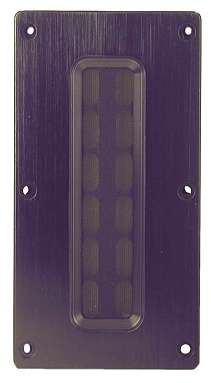## OmnesAudio MT 2.01

order no. not-available- not available

ribbon tweeter.

• frequency range = 1200-30000 Hz
• resonance frequency fs = 1500 Hz
• impedance R = 8 Ohm
• sound pressure level SPL = 92 dB (2,83V; 1m)
• DC resistance Re = 7,6 Ohm
• mounting depth (not countersunk) t = 22 mm
PDF data sheet## OmnesAudio T 25 Ti

order no. not-available- not available

dome tweeter with high quality dome. The voice coil windings are immersed in magnetic fluid to increase short term power handling capacity, to reduce the compression and the Q factor..

• resonance frequency fs = 1700 Hz
• impedance R = 4 Ohm
• sound pressure level SPL = 93 dB (2,83V; 1m)## OmnesAudio T 2.01

order no. not-available- not available

25 mm tweeter with silk cone.

• frequency range = 2500-20000 Hz
• resonance frequency fs = 1500 Hz
• impedance R = 8 Ohm
• sound pressure level SPL = 90 dB (2,83V; 1m)
• voice coil diameter = 25 mm
• mounting diameter d = 72 mm
• overall diameter d = 99,5 mm
• mounting depth (not countersunk) t = 35 mm
PDF data sheet

The cabinet volume calculations are executed considering an external resistance of 0.3 Ohm. We are using, if no other data is known or available, the information supplied by the manufacturer.

Please note: the frequency response doesn't say much about the cut-off frequency inside the cabinet! Therefore, we mostly give you 2 examples with dimensioning considerations and an indication, how low the loudspeaker will reproduce.

Home